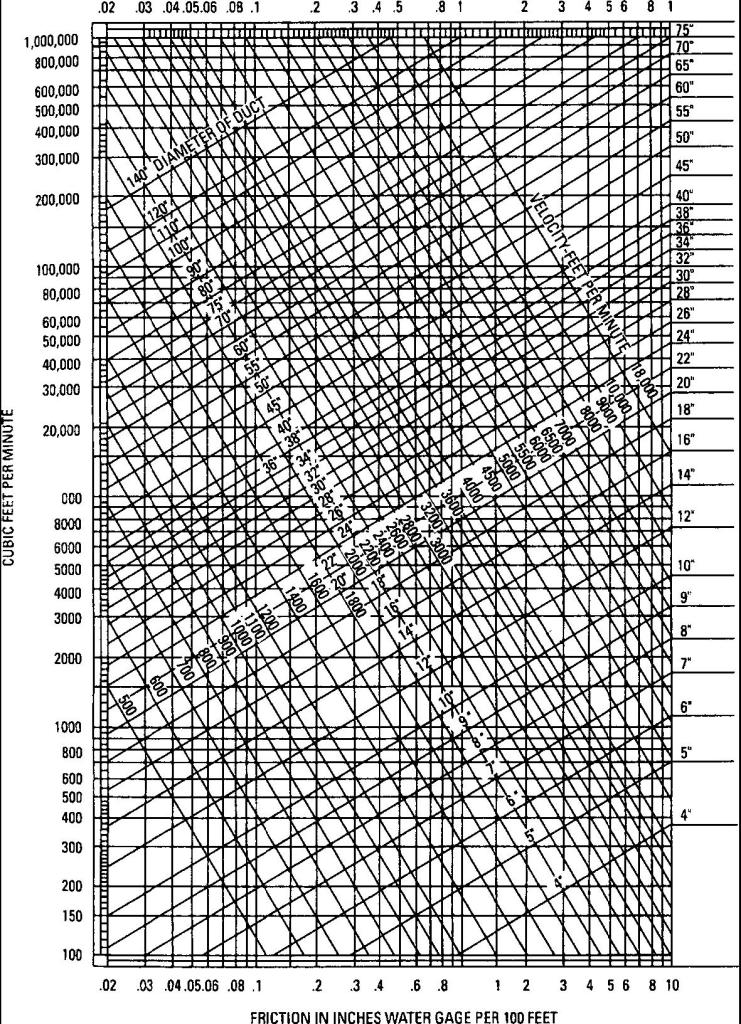# Resistance Losses in HVAC Duct Systems

In general, it can be said that duct sizes and the depth of a duct in particular are affected by the available space in the building. For this reason, although the round duct is the most economical shape from the standpoint of friction per unit area and from the standpoint of metal required for construction per unit of area, it is rarely possible, except in industrial buildings, to use round ducts largely. Square duct is the preferable shape among those of rectangular cross section. Headroom limitations usually require that the duct be flattened.

To illustrate the use of charts when sizing a duct system, consider Example below.

Example 3-2 Assume a system requiring the delivery of 5000 ft3/min. The distribution requirement is the movement of the entire volume a distance of approximately 80 ft, with the longest branch beyond that point conveying 1000 ft3/min for an additional 70 ft. Assume further that the operating characteristics of the fan and the resistance of the coils, filters, and so on, allow a total supply duct resistance of 0.10-in. water-gage resistance pressure. The supply duct is not to be more than 12 in. deep.

solution The total length of the longest run is 80 + 70 = 150 ft:

100/150 =0.10=0.067 in.water gage

Starting at this resistance at the bottom of Fig. 3-27, follow upward to the horizontal line representing 5000 ft3/min. At this point, read the equivalent size of round duct required that is approximately 28 in. in diameter. Move diagonally upward to the right on the 28-in.-diameter line, and then across horizontally on this line in Fig. 3-27 to the vertical line representing the 12-in. side of a rectangular duct. At this point, read 60 in. as the width of the rectangular duct required on the intersecting curve line.

Thus, for the main duct run, the duct size will be 60 × 12 in. For the branch conveying 1000 ft3/min, from the point where the 0.067-in. resistance line intersects the 1000-ft3/min line, read 16 in. as the equivalent round duct required. Following through on Fig. 3-27 as for a larger duct, read 12 × 18 in. as the size of the branch duct.

Duct runs should take into account the number of bends and offsets. Obstructions of this kind are usually represented in terms of the equivalent length of straight duct necessary to produce the same resistance value. Where conditions require sharp or right-angle bends, vane elbows composed of a number of curved deflectors across the air-stream should be used.Figure 3-27 Graphic representation of air-duct areas.